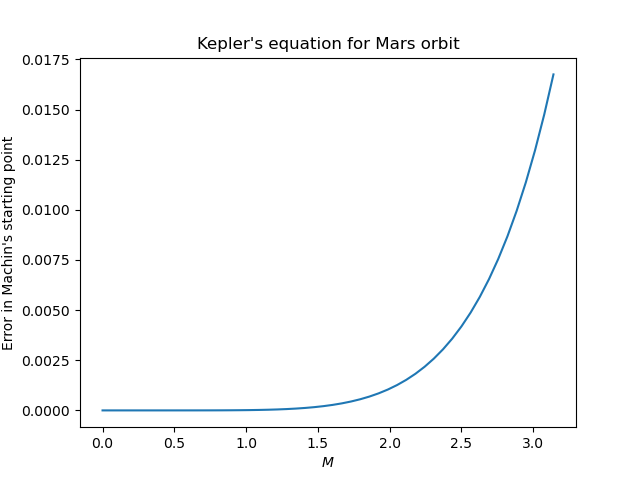# Python code to solve Kepler’s equation

The previous post looked at solving Kepler’s equation using Newton’s method. The problem with using Newton’s method is that it may not converge when the eccentricity e is large unless you start very close to the solution. As discussed at the end of that post, John Machin came up with a clever way to start close. His starting point is defined as follow.The variable s is implicitly defined as the root of a cubic polynomial. This could be messy. Maybe there are three real roots and we have to decide which one to use. Fortunately this isn’t the case.

The discriminant of our cubic equation is negative, so there is only one real root. And because our cubic equation for s has no s² term the expression for the root isn’t too complicated.

Here’s Python code to solve Kepler’s equation using Newton’s method with Machin’s starting point.

    from numpy import sqrt, cbrt, pi, sin, cos, arcsin, random

# This will solve the special form of the cubic we need.
def solve_cubic(a, c, d):
assert(a > 0 and c > 0)
p = c/a
q = d/a
k = sqrt( q**2/4 + p**3/27 )
return cbrt(-q/2 - k) + cbrt(-q/2 + k)

# Machin's starting point for Newton's method
# See johndcook.com/blog/2022/11/01/kepler-newton/
def machin(e, M):
n = sqrt(5 + sqrt(16 + 9/e))
a = n*(e*(n**2 - 1)+1)/6
c = n*(1-e)
d = -M
s = solve_cubic(a, c, d)
return n*arcsin(s)

def solve_kepler(e, M):
"Find E such that M = E - e sin E."
assert(0 <= e < 1)
assert(0 <= M <= pi)
f = lambda E: E - e*sin(E) - M
E = machin(e, M)
tolerance = 1e-10

# Newton's method
while (abs(f(E)) > tolerance):
E -= f(E)/(1 - e*cos(E))
return E


To test this code, we’ll generate a million random values of e and M, solve for the corresponding value of E, and verify that the solution satisfies Kepler’s equation.

    random.seed(20221102)
N = 1_000_000
e = random.random(N)
M = random.random(N)*pi
for i in range(N):
E = solve_kepler(e[i], M[i])
k = E - e[i]*sin(E) - M[i]
assert(abs(k) < 1e-10)
print("Done")


All tests pass.

Machin’s starting point is very good, and could make an adequate solution on its own if e is not very large and if you don’t need a great deal of accuracy. Let’s illustrate by solving Kepler’s equation for the orbit of Mars with eccentricity e = 0.09341.Here the maximum error is 0.01675 radians and the average error is 0.002486 radians. The error is especially small for small values of M. When M = 1, the error is only 1.302 × 10-5 radians.